«上一篇文章快速检索 高级检索

 智能系统学报2019, Vol. 14Issue (5): 1017-1025  DOI: 10.11992/tis.2018090240

### 引用本文QIN Ya, SHEN Guowei, YU Hongxing. Large-scale network security entity recognition method based on Hadoop[J]. CAAI Transactions on Intelligent Systems, 2019, 14(5): 1017-1025. DOI: 10.11992/tis.201809024.### 文章历史

1. 贵州大学 计算机科学与技术学院，贵州 贵阳 550025;
2. 贵州大学 贵州省公共大数据重点实验室，贵州 贵阳 550025

Large-scale network security entity recognition method based on Hadoop
QIN Ya 1,2, SHEN Guowei 1,2, YU Hongxing 1,2
1. Department of Computer Science and Technology, Guizhou University, Guiyang 550025, China;
2. Guizhou Provincial Key Laboratory of Public Big Data, Guizhou University, Guiyang 550025, China
Abstract: In this era of big data, a fundamental problem for constructing network security knowledge graphs is how to efficiently and accurately identify the network security entities present in multi-source heterogeneous data. This study focuses on text data related to network safety and investigate the use of a security entity recognition algorithm that supports massive-network text data, thereby laying a foundation for building the network security knowledge graph. To efficiently and accurately extract the security entities in massive-network text data, we propose an improved conditional random fields (CRF) algorithm based on the Hadoop distributed computing framework to segment data sets effectively, which realize efficient and accurate recognition of security entities. The experimental results reveal that the proposed security entity recognition algorithm achieved a high precision rate on a large-scale real network data set and improved the efficiency of network security entity recognition..
Key words: big data    heterogeneous data    network security    knowledge graph    security entity    entity recognition    network data    Hadoop    CRF algorithm

1)网络安全实体数量众多且类型多种多样，难以满足自然语言处理领域中的命名实体定义，且不断地会有未登录词作为新的安全实体出现。

2)网络文本数据中的实体具有不同的结构，比如网络安全实体出现大量的嵌套、别名、缩略词等问题，没有严格的构词规律可以遵循。

3)在大规模数据条件下，基于机器学习模型的算法运行效率较低，单机上的安全实体识别算法难以满足安全实体识别需求。

2)分析网络安全数据中的实体结构特征，给出了网络安全实体识别规则，并进一步提出了改进的CRF算法，对算法进行分析。

3)在真实的数据集上，针对提出的网络安全实体识别方法，结合评测标准进行对比实验，结果表明本文提出的方法在准确率和效率上都有所提高。

1 问题定义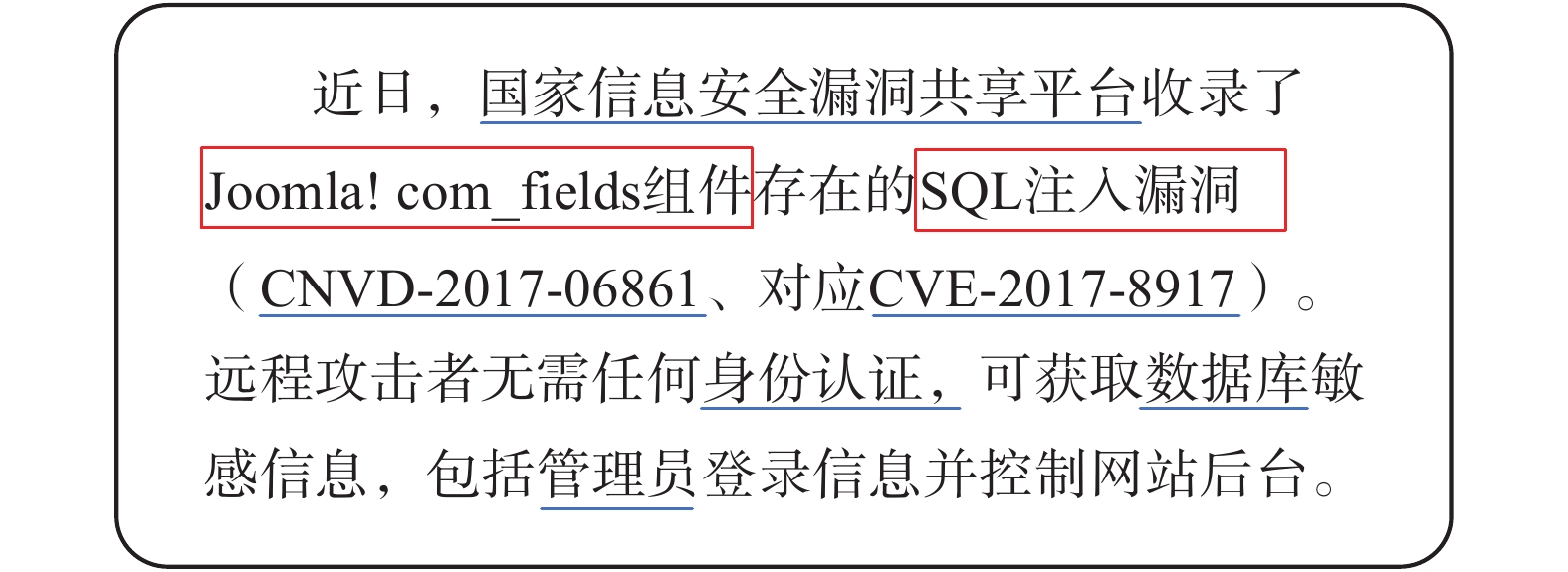Download: 图 1 Web文本数据中的安全实体识别 Fig. 1 Security entity recognition in web text data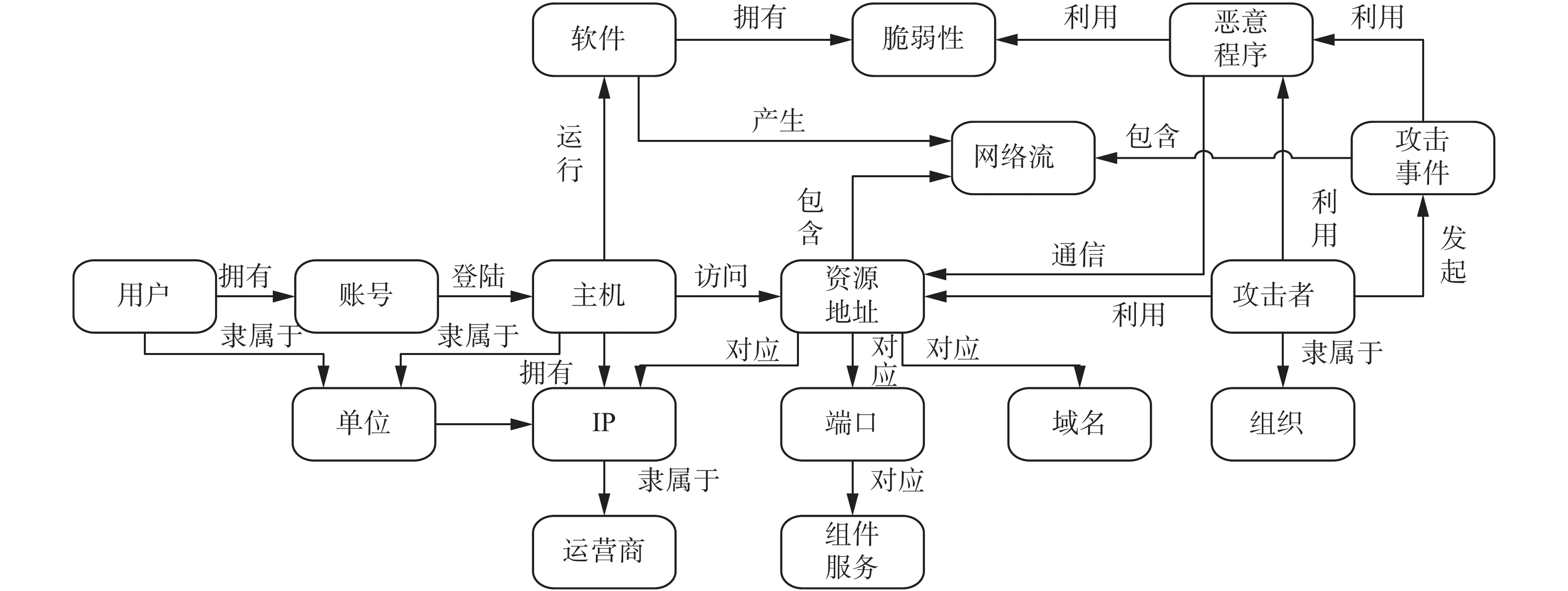Download: 图 2 网络安全实体的本体模型 Fig. 2 Ontological model of network security entity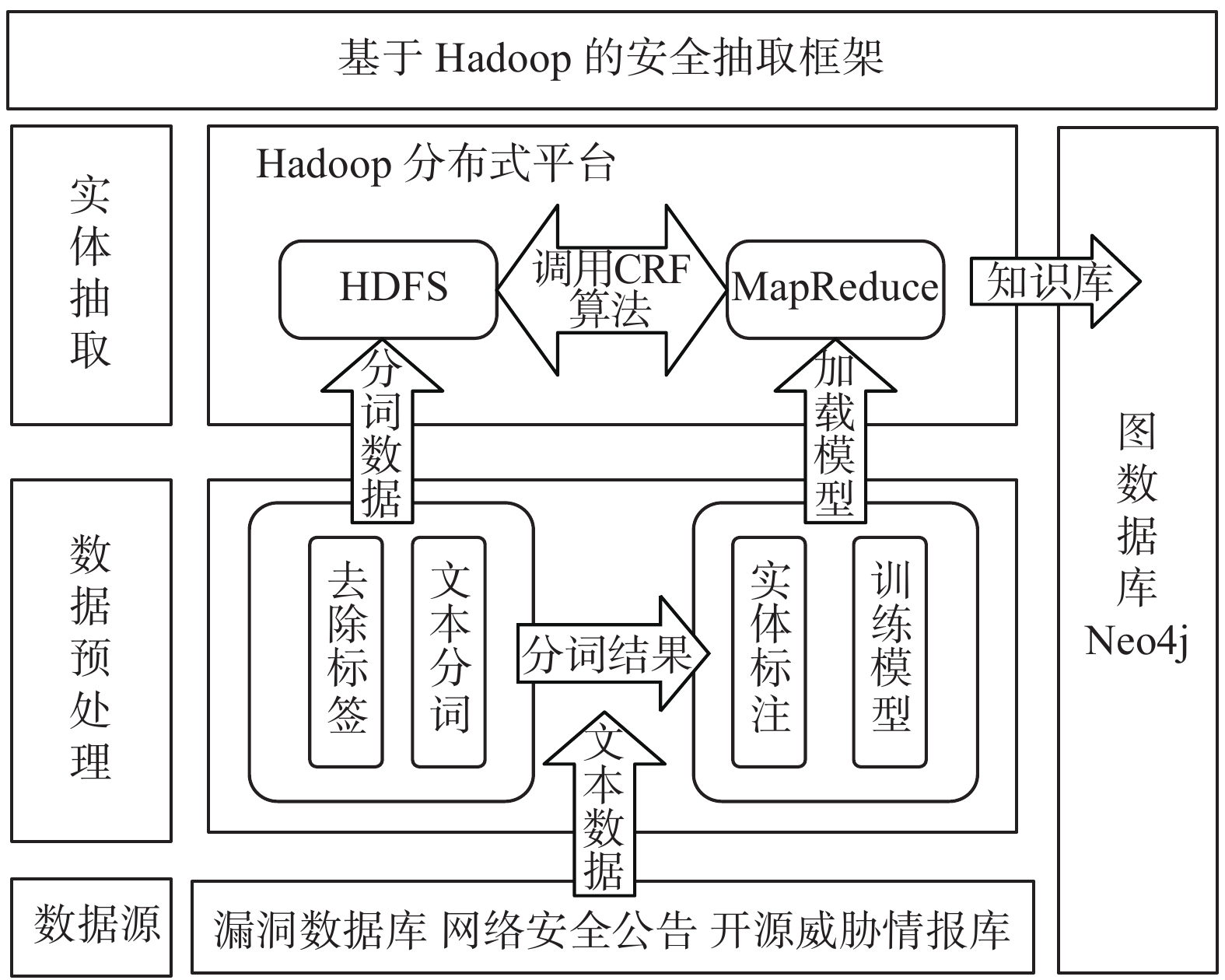Download: 图 3 网络安全实体识别框架 Fig. 3 Network security entity recognition framework

1)数据预处理

①使用正则表达式对网页文本进行预处理，去除网页中的关于HTML的标签。

②通过使用Stanford CoreNLP提供的分词工具，将去除标签后的文本数据进行分词。

③构建语料库，由于网络安全领域没有统一的语料库，因此在对安全实体识别前，需要对其构建语料库。对已经分词的文本数据进行实体标注，特征实体时，可以通过程序先将所有实体标注为OO表示未识别实体；然后进行网络安全实体标注，由人工判断手动标注为EnEn表示安全实体。

④最后，训练网络安全实体模型。在训练过程中，根据训练工具的格式要求将前面的所有标注后的数据转化成特定的数据格式，然后利用CRF算法进行模型训练。

2)中文网络安全实体识别

 ${\rm{Map}}: < {K_1},{V_1} > \to < {\rm{list}}({K_2},{V_2}) >$ (1)
 $\operatorname{Re} {\rm{duce}}: < {K_2},{\rm{list}}({V_2}) > \to < {K_3},{V_3} >$ (2)

1)调用Map函数；

2)对数据D中的文档进行分词并标注，形成观察序列 ${D_i} = {\rm{\{ }}{x_1},{x_2},\cdots ,{x_{{M_i}}}{\rm{\} }}$

3)CRFClassifier(Di) //调用CRF算法识别网络安全实体；

4)经过模型预测形成标注序列 $Y = {\rm{\{ }}{y_1},{y_2},\cdots {y_i},$ ${y_{M_i}}\}$

5)调用Reduce函数；

6)根据标注序列进行分类，得到K类实体的 $E = \{ {E_1},{E_2},\cdots ,{E_K}\}$

7)根据平均值获得最终的 ${E_i} = \{ e_i^1,e_i^2,\cdots ,e_i^{{M_i}}\}$

8)EntityStore.CreateNeo4j(key)//存储到Neo4j

3.2 CRF算法描述

 $p({{{Y}}_v}|{{X}},{{{Y}}_w},w \ne v) = p({{{Y}}_v}|{{X}},{{{Y}}_w},w \sim v)$ (3)

 $p(y|x,\lambda )\infty \exp (\sum\limits_{i,j} {{\lambda _j}} {t_j}({y_{i - 1}},{y_i},x,i) + \sum\limits_{i,k} {{u_k}{s_k}({y_i},x,i)} )$ (4)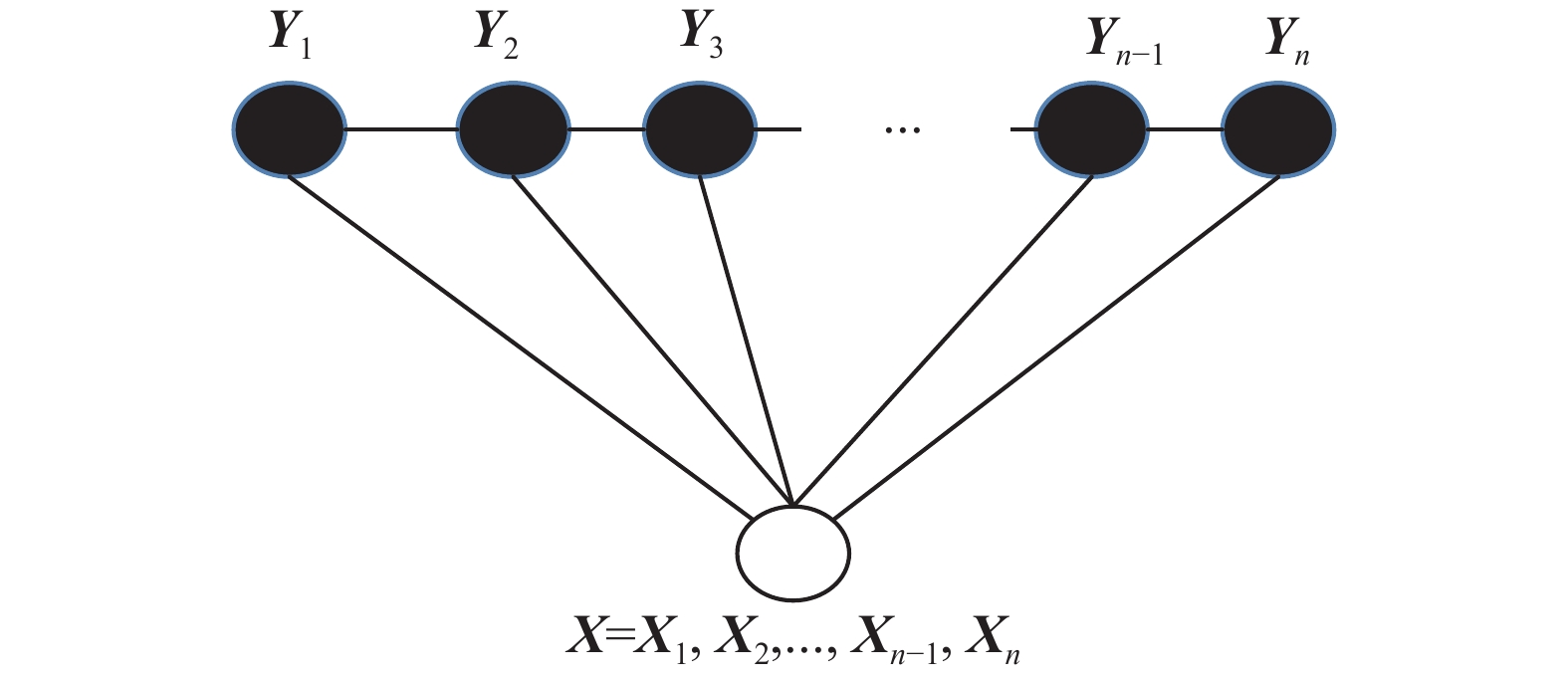Download: 图 4 链式条件随机场 Fig. 4 Chain conditional random field

 $p(y|x,{\textit{λ}}) = \frac{1}{{Z(x)}}\exp (\sum\limits_{j = 1}^j {\sum\limits_{i = 1}^n {{{\textit{λ}} _j}{f_j}({y_{i - 1}},{y_i},x,i))} }$ (5)
 $Z(x) = \sum\limits_y {\exp (\sum\limits_{j = 1}^j {\sum\limits_{i = 1}^n {{{\textit{λ}} _j}{f_j}({y_{i - 1}},{y_i},x,i)} } )}$ (6)

 $f_j({y_{i - 1}},{y_i},x,i) = \left\{ \begin{array}{l} b(x,i) ,\begin{array}{*{20}{c}} {} \end{array}{y_{i - 1}} = < {\rm{titile}} > ,{y_i} = < {\rm{author}} > \\ 0,\quad{\text{其他}} \end{array} \right.$ (7)

 $\frac{{\partial L({\textit{λ}})}}{{\partial{{\textit{λ}}_j}}} = {E_{\beta (x,y)}}{f_j}(x,y) - \sum {{E_{p(y|{x^k},{\textit{λ}})}}{f_j}({x^{(k)}},y) - \frac{{{{\textit{λ}}_k}}}{{{\sigma ^2}}}}$ (8)

4 实验及分析 4.1 实验环境及数据集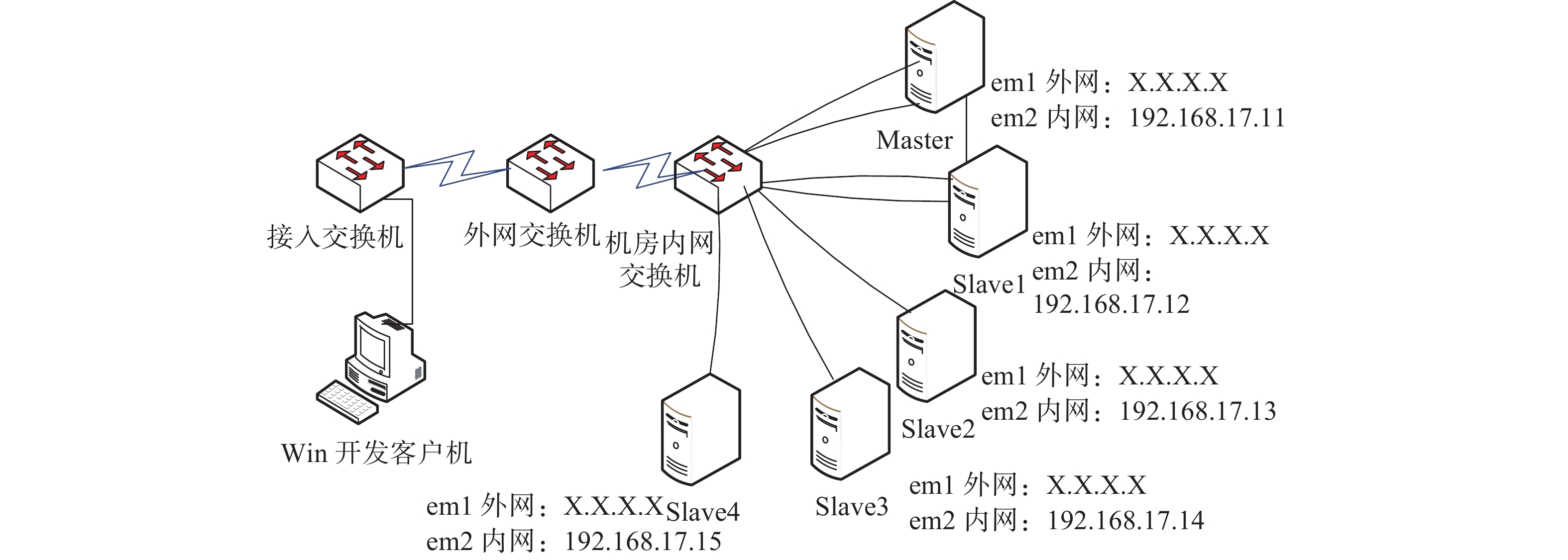Download: 图 5 Hadoop平台的拓扑结构 Fig. 5 Topological diagram of the Hadoop platform表 1 服务器的硬件配置 Tab.1 Server hardware configuration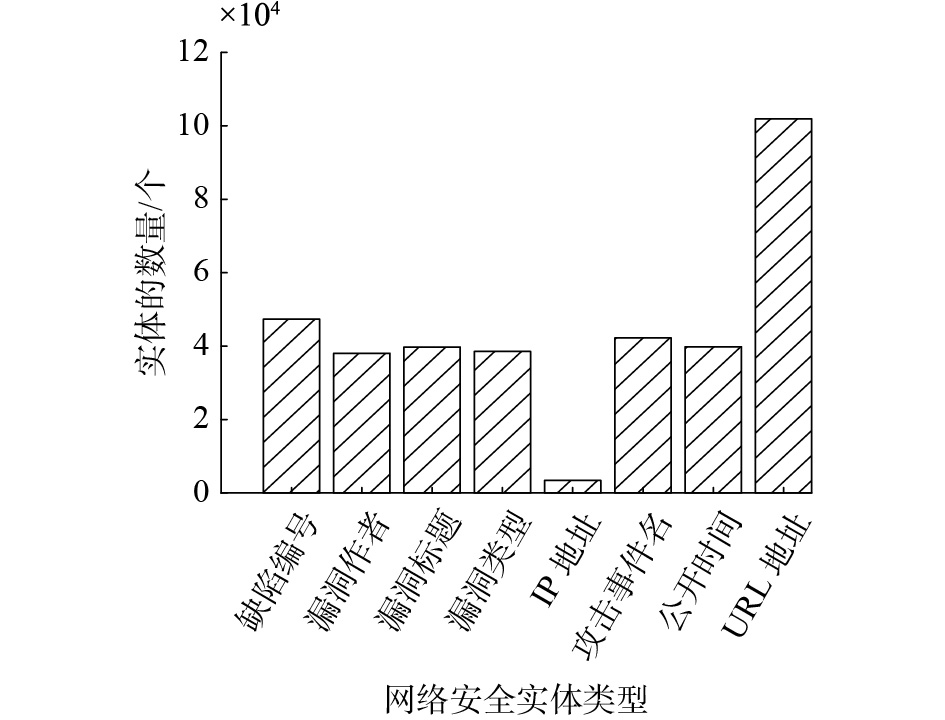Download: 图 6 语料库统计信息 Fig. 6 Network security entity types
4.2 小规模识别率对比实验

 $P = \frac{{{N_2}}}{{{N_1}}}$ (9)

 $R = \frac{{{N_2}}}{N}$ (10)

 $F = \frac{{2 \times P \times R}}{{P + R}}$ (11)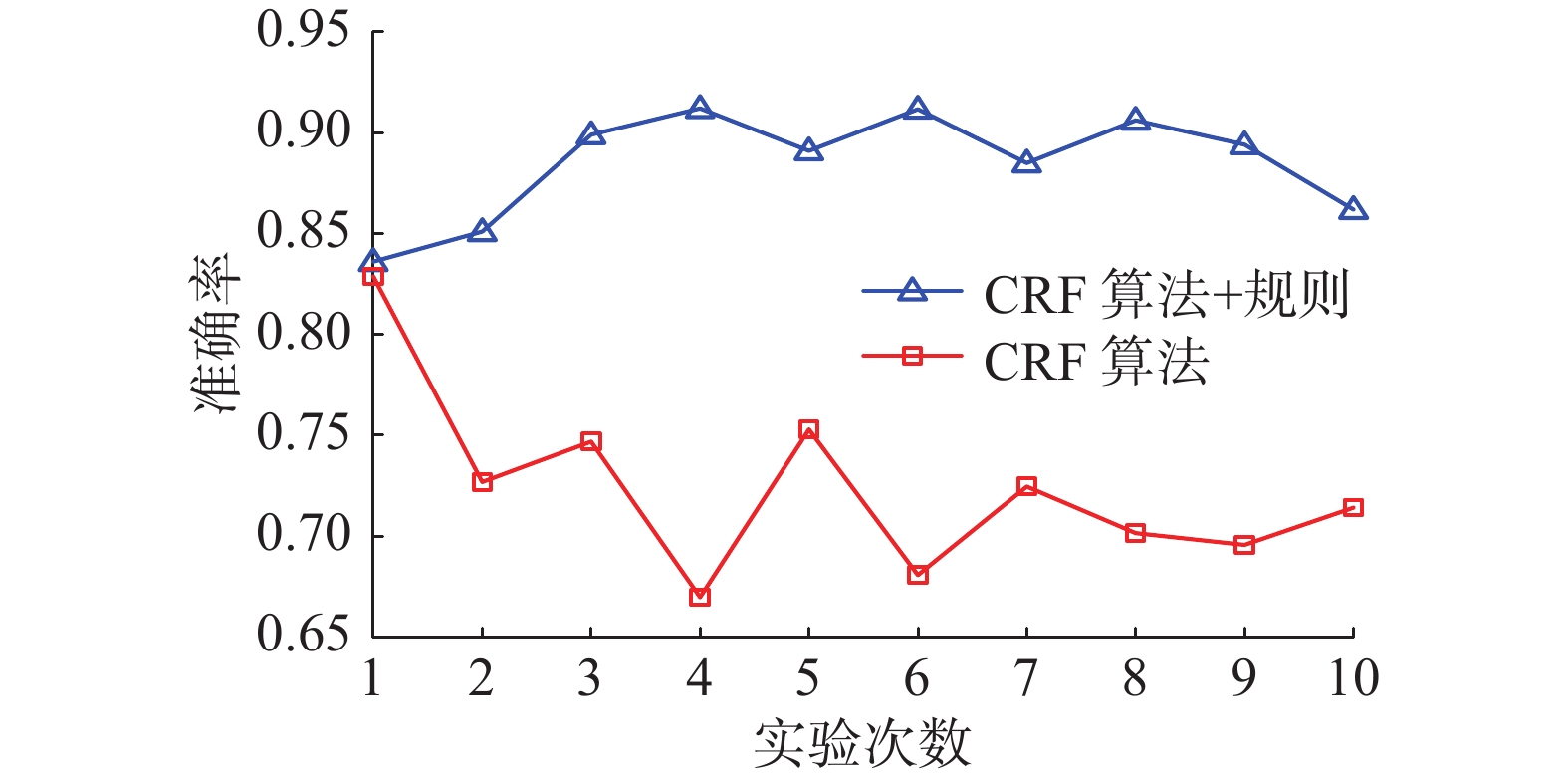Download: 图 7 准确率对比结果 Fig. 7 Comparisons of precision of results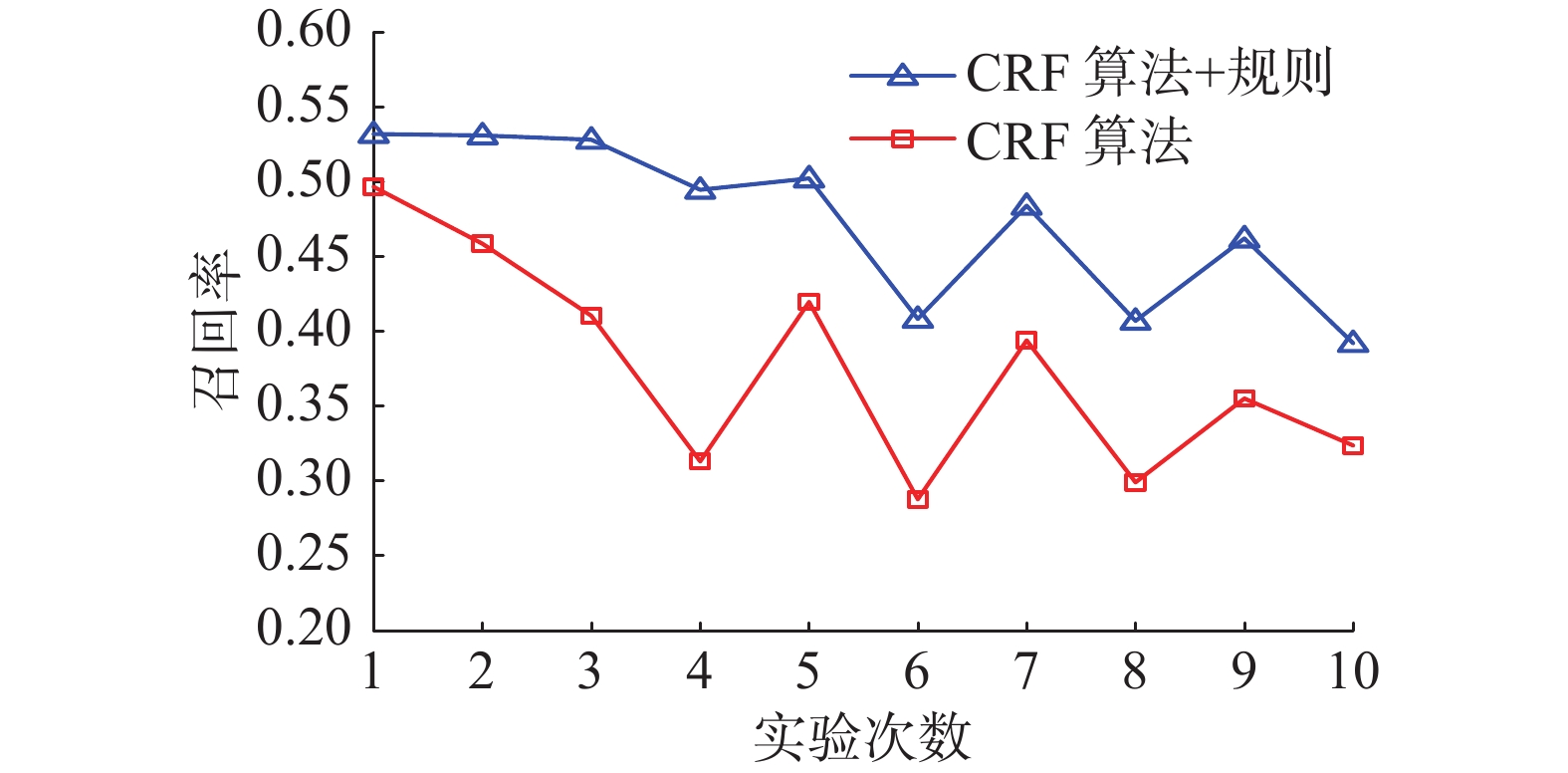Download: 图 8 召回率对比结果 Fig. 8 Comparisons of recall results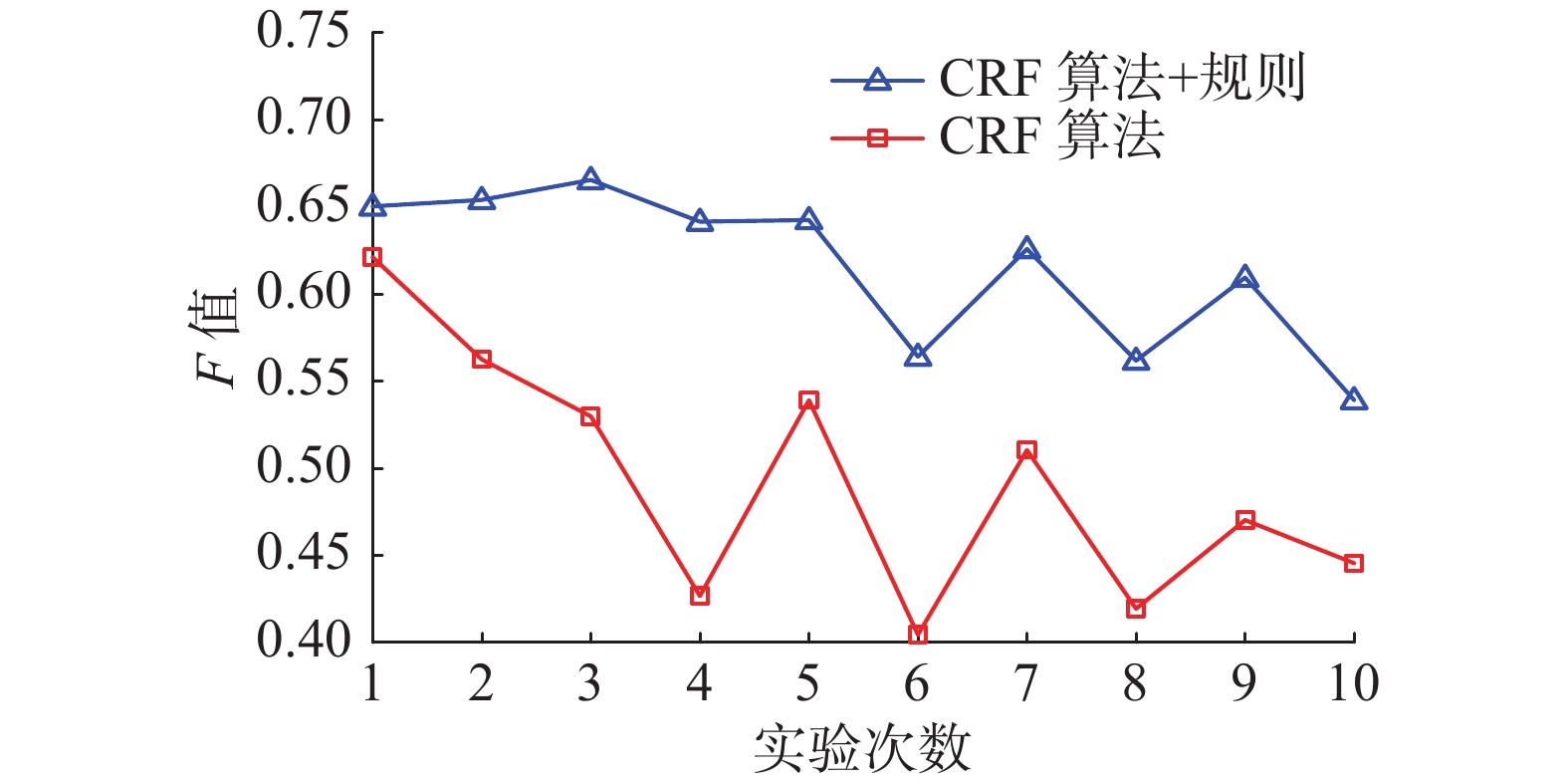Download: 图 9 F值对比结果 Fig. 9 Comparisons of F-value results

4.3 大规模对比实验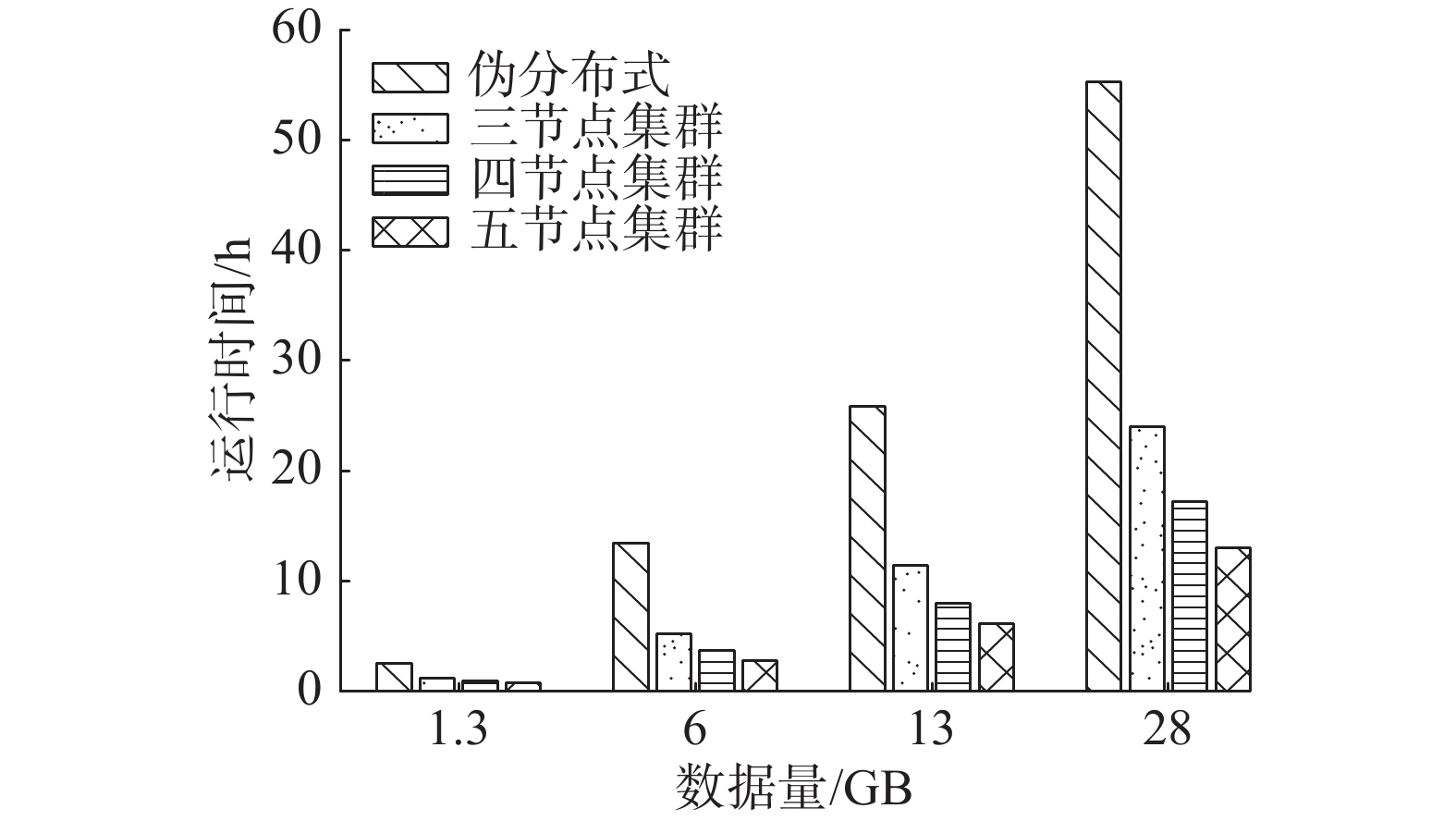Download: 图 10 不同节点数下的运行时间对比结果 Fig. 10 Comparison of running times for different node numbers

4.4 算法的可扩展性分析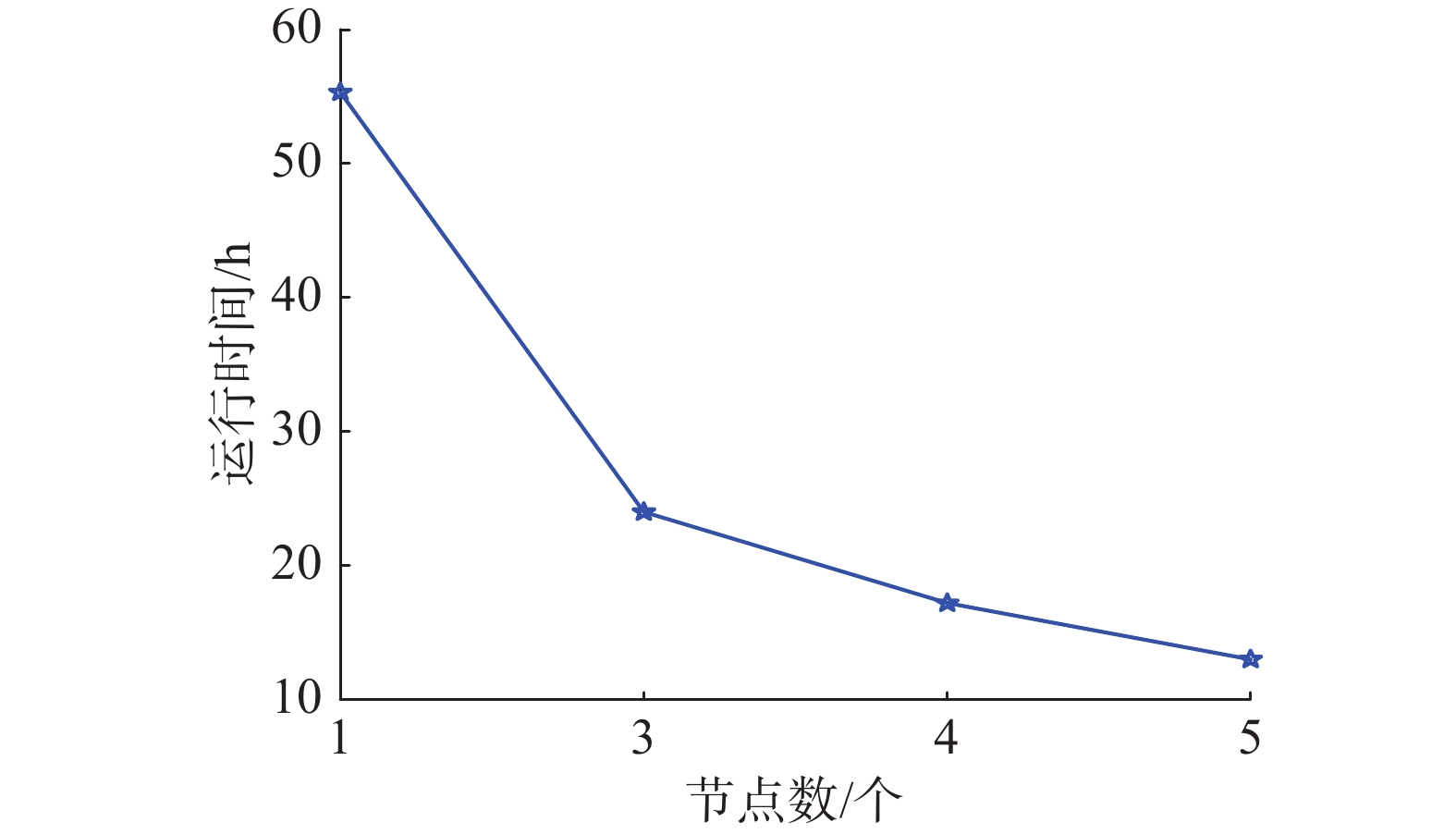Download: 图 11 28 GB数据运行时间对比 Fig. 11 Comparison of 28 GB data running times
4.5 网络安全实体识别实例分析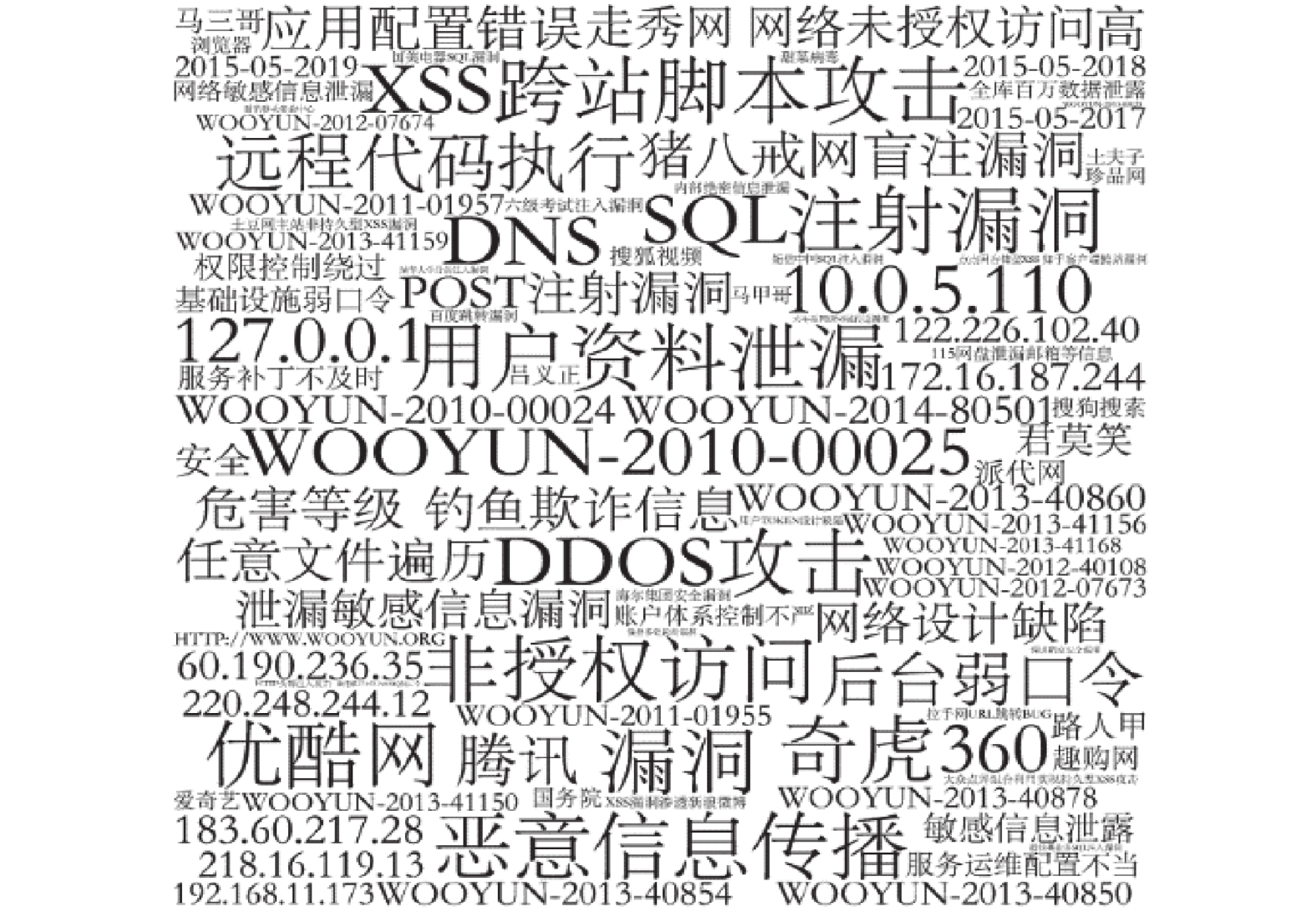Download: 图 12 网络安全实体词云图 Fig. 12 Word cloud map of network security entity
5 结束语

  廖建新. 大数据技术的应用现状与展望[J]. 电信科学, 2015, 31(7): 1-12. LIAO Jianxin. Big data technology: current applications and prospects[J]. Telecommunications science, 2015, 31(7): 1-12. (0)  单琳. 网络威胁情报发展现状综述[J]. 保密科学技术, 2016(8): 28-33. SHAN Lin. Overview of the development status of cyber threat intelligence[J]. Security science and technology, 2016(8): 28-33. (0)  南湘浩, 陈钟. 网络安全技术概论[J]. 计算机安全, 2003(30): 76. NAN Xianghao, CHEN Zhong. Introduction to network security technology[J]. Computer security, 2003(30): 76. (0)  陈兴蜀, 曾雪梅, 王文贤, 等. 基于大数据的网络安全与情报分析[J]. 工程科学与技术, 2017, 49(3): 1-12. CHEN Xingshu, ZENG Xuemei, WANG Wenxian, et al. Big data analytics for network security and intelligence[J]. Advanced engineering sciences, 2017, 49(3): 1-12. (0)  张晓艳, 王挺, 陈火旺. 命名实体识别研究[J]. 计算机科学, 2005, 32(4): 44-48. ZHANG Xiaoyan, WANG Ting, CHRN Huowang. Research on named entity recognition[J]. Computer science, 2005, 32(4): 44-48. DOI:10.3969/j.issn.1002-137X.2005.04.014 (0)  JONES C L, BRIDGES R A, HUFFE K M T, et al. Towards a relation extraction framework for cyber-security concepts[C]//Proceedings of the 10th Annual Cyber and Information Security Research Conference. Oak Ridge, USA, 2015: 11. (0)  JOSHI A, LAL R, FININ T, et al. Extracting cybersecurity related linked data from text[C]//Proceedings of 2013 IEEE Seventh International Conference on Semantic Computing. Irvine, USA, 2013: 252–259. (0)  LAL R. Information extraction of security related entities and concepts from unstructured text[D]. Baltimore County: University of Maryland, 2013. (0)  MULWAD V, LI Wenjia, JOSHI A, et al. Extracting information about security vulnerabilities from web text[C]//Proceedings of 2011 IEEE/WIC/ACM International Conferences on Web Intelligence and Intelligent Agent Technology. Lyon, France, 2011: 257–260. (0)  翟菊叶, 陈春燕, 张钰, 等. 基于CRF与规则相结合的中文电子病历命名实体识别研究[J]. 包头医学院学报, 2017, 33(11): 124-125, 130. ZHAI Juye, CHEN Chunyan, ZHANG Yu, et al. A study on the named entity recognition of Chinese electronic medical record based on combination of CRF and rules[J]. Journal of Baotou Medical College, 2017, 33(11): 124-125, 130. (0)  张晓艳, 王挺, 陈火旺. 基于混合统计模型的汉语命名实体识别方法[J]. 计算机工程与科学, 2006, 28(6): 135-139. ZHANG Xiaoyan, WANG Ting, CHEN Huowang. A mixed statistical model-based method for chinese named entity recognition[J]. Computer engineering and science, 2006, 28(6): 135-139. DOI:10.3969/j.issn.1007-130X.2006.06.043 (0)  徐梓豪. 基于统计模型的中文命名实体识别方法研究及应用[D]. 北京: 北京化工大学, 2017. XU Zihao. Statistical model based Chinese named entity recognition methods and its application to medical records[D]. Beijing: Beijing University of Chemical Technology, 2017. (0)  RABINER L R. A tutorial on hidden Markov models and selected applications in speech recognition[M]//WAIBEL A, LEE K F. Readings in Speech Recognition. San Francisco: Morgan Kaufmann, 1990: 267–296. (0)  KOELING R. Chunking with maximum entropy models[C]//Proceedings of the 2nd Workshop on Learning Language in Logic and the 4th Conference on Computational Natural Language Learning. Lisbon, Portugal, 2000: 139–141. (0)  郑秋生, 刘守喜. 基于CRF的互联网文本命名实体识别研究[J]. 中原工学院学报, 2016, 27(1): 70-73, 95. ZHRNG Qiusheng, LIU Shouxi. Research of web text named entity recognition based on CRF[J]. Journal of Zhongyuan University of Technology, 2016, 27(1): 70-73, 95. DOI:10.3969/j.issn.1671-6906.2016.01.017 (0)  朱颢东, 杨立志, 丁温雪, 等. 基于主题标签和CRF的中文微博命名实体识别[J]. 华中师范大学学报(自然科学版), 2018, 52(3): 316-321. ZHU Haodong, YANG Lizhi, DING Wenxue, et al. Named entity recognition of Chinese microblog based on theme tag and CRF[J]. Journal of Central China Normal University (Natural Sciences), 2018, 52(3): 316-321. (0)  TELNOV Y, SAVICHEV I. Ontology-based competency management: infrastructures for the knowledge intensive learning organization[C]//Proceedings of the 1st International Early Research Career Enhancement School. Cham, Switzerland, 2016: 249–256. (0)  IANNACONE M, BOHN S, NAKAMURA G, et al. Developing an ontology for cyber security knowledge graphs[C]//Proceedings of the 10th Annual Cyber and Information Security Research Conference. Oak Ridge, USA, 2015: 12. (0)  DEAN J, GHEMAWAT S. MapReduce: simplified data processing on large clusters[C]//Proceedings of the 6th Conference on Symposium on Opearting Systems Design and Implementation. San Francisco, USA, 2004: 10. (0)  LAFFERTY J D, MCCALLUM A, PEREIRA F C N. Conditional random fields: probabilistic models for segmenting and labeling sequence data[C]//Proceedings of the 18th International Conference on Machine Learning. Williamstown, USA, 2001: 282–289. (0)